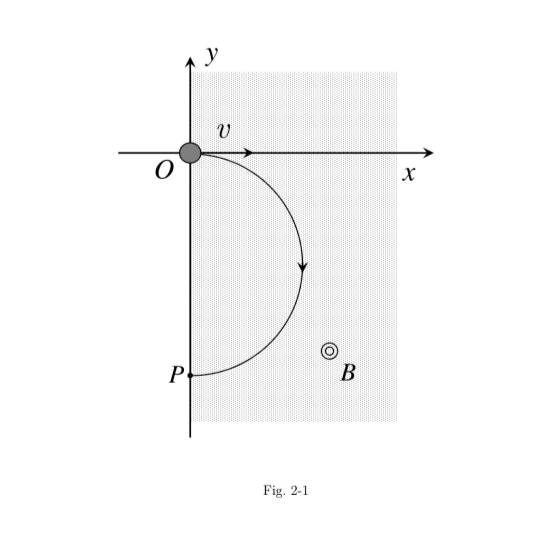# How to interpret magnetic field diagrams?

• Dan13

#### Dan13

Homework Statement
The right half of the x-y plane is filled with a uniform magnetic field of
magnitude B pointing out of the page as shown in Fig. 2-1. A charged
particle of mass m enters the magnetic field region along the negative x axis
in the positive x direction with speed v. The magnitude of the charge is
denoted by q. The trajectory of the particle describes a semi-circle as shown
in Fig. 2-1. Answer the following questions.
(1) Is the charge of the particle positive or negative?
(2) Find the magnitude of the force on the particle.
(3) Find the radius of the circular orbit.
(4) Find the time it takes the particle to travel from O to P.
Relevant Equations
1) I'l have and idea to determinate the charge of this particle using the rule of the right or left hand but I'm having trouble interpreting the diagram to fir this rules in order to determinate the charge but I'm not able to do it.
2) I have a problem here I think there is no force here because as I see the image there's is a angle of 0 between the particle anthems magnetic field and due to F=qvSenθ there's no force.
3) I've tried to rearrange the formula of force and speed but haven't achieved something.
4) Here I have no idea about how to solve this.
I'd be grateful if some one help me to solve this I've tried to investigate and read but I haven't found the topics also if you could be so kind of leaving the topics of this problems or any resources it would be great a list for me to investigate#### Attachments

I will tell you things and you can ask questions.
The symbol next to the B field is the tip of an arrow meaning B points out of the page at you.

•Dan13
Have you tried looking in the textbook (or course website)?

Have you tried looking in the textbook (or course website)?
I tried in the tutorial and formulay section but I just find the equation for the radius but I'd like to know where does it come I also found information for the force in the particule as I wrote in the relevant equation section but nothing related with a diagram like this, and that's my real problem here I can't interpret this diagram I can't find how useful the diagram would be

Can you find an equation that relates the electron motion and the magnetic field direction to the force on the electron? (Note: all are vectors)